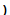Vertex of a Parabola - Maple Help

Vertex of a Parabola

Main Concept

The vertex of a parabola is the point at the intersection of the parabola and its line of symmetry.

For a parabola whose equation is given in standard form , the vertex will be the minimum (lowest point) of the graph if $a>0$ and the maximum (highest point) of the graph if $a<0$.Vertex form

The vertex form of a parabola is   where $\left(h,k\right)$ is the vertex. The variable $a$ has the same value and function as the variable in the standard form. If  (positive)  the parabola opens up, and if (negative) the parabola opens down.

The vertex form of a parabola is usually not provided. To convert from the standard form  to vertex form, you must complete the square.Example of completing the square:

 1. Factor the leading coefficient out of the first two terms. 2. Complete the square by addition and subtracting the magic number - the square of half the coefficient of $x$. $=$ 3. Factor out the constant . $=$ 4. Factor the perfect square. $=$

Use the sliders to change the vertex $\left(h\mathit{,}k\right)$ and observe how your changes affect the graph of the parabola.

Observe that when the graph opens up, the range of the corresponding quadratic function is $\left[k\mathit{,}\mathit{\infty }\right)$, while if it opens down, the range is $\left(\mathit{-}\mathit{\infty }\mathit{,}k\right]$.

 Value of $a$Value of $k$:Value of $h$:More MathApps